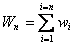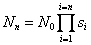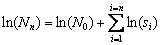### N. Thompson Hobbs and Ray Hilborn. 2006. Alternatives to statistical hypothesis testing in ecology: a guide to self teaching. Ecological Applications 16:5–19.

Appendix B. What likelihood to use?

Likelihood is the gateway to a range of tools including maximum likelihood estimation, likelihood profile, model selection via information theoretics, and Bayesian analysis. Perhaps the hardest step for someone attempting to learn to use likelihood theory is deciding what likelihood to use.  In this box, we will provide some guidelines on how to choose the appropriate likelihood function for your problem.

What are the options?

Remembering that likelihood is proportionate to probability (Eq. 1 in main paper), likelihood functions are drawn from the range of probability functions for counts and probability density functions for continuous data.  Essentially you must decide what the underlying probability model is for the data, and this will dictate the likelihood to use.  For convenience we will refer to both of these functions as pdfs.  In our experience, the most commonly used pdfs in ecology, in rough order of frequency of use, are normal, lognormal, Poisson, binomial, multinomial, negative-binomial, beta, and gamma.  The first five can be thought of as the standard elements of an ecologist’s tool kit and probably account for 95% of the probability functions used in ecological papers.  As ecologists look more clearly at the ecological processes, we suspect the negative-binomial, beta, and gamma distributions will increase in use.

These pdfs can be divided into two groups, those that deal with data that are measured as a continuous variable on the real number line, normal, lognormal, beta and gamma, and those that deal with counts, Poisson, binomial, multinomial, and the negative binomial. The division is often easy to make; measurements of weight, growth, density, etc., naturally fall into the continuous category, and the normal and lognormal immediately arise as alternates.  Similarly, if the data are counts of individuals, as in those surviving from a tagging experiment, or counts of individuals per quadrat or transect, etc., then the Poisson, binomial, and multinomial are appropriate.  Here, we will not go into the details of each of these functions, but instead refer the reader to a more thorough treatment in Hilborn and Mangel (1997).  However, we will focus attention on how to decide which pdf to use.

There are two ways to choose which pdfs to use as likelihood functions, either by a theoretical understanding of the data, or empirically by evaluating how well the data fit alternative likelihoods.

Theory: How do the data arise?

The foundation of the normal and log normal distributions is the “central limit theorem” that demonstrates that the sum of a series of independent random numbers will be normally distributed if the number of random variables is large.  Thus, any process that can be thought of as being the result of the sum of processes will usually be normally distributed.  For instance, if we think of the weight of an organism as the result of a series of daily growth increments, then we would expect weight to be normally distributed.  This could be written aswhere Wn is the weight of an organism at age n, and wi is the weight gained at time i.  The central limit theorem is the reason that we expect many population characteristics, such as average size, weight, etc., to be normally distributed, because the population characteristic is the sum of a series of random events associated with the trait for each individual in the population or sample from the population.

Often the process is multiplicative instead of additive, as in the case of survival.  The number of individuals in a cohort surviving to a specific age would be written as, where Nn is the number surviving to age n, N0 is the number born, and si, is the survival during a time period (day or year) i.  Because this can be rewritten as a sum of logarithms,, we can expect the log of N to be normally distributed or N to be lognormally distributed.

For count data, the binomial and multinomial are used when the counts can fall into two (binomial) or more (multinomial classes).  In ecology, these distributions are commonly used for analysis of mark-recapture data (many classes of when or where tagged individuals are recovered) or in the analysis of age, size, or sex distribution data (the number of individuals measured in each class is counted).

The Poisson distribution is generally used where the data are simply the number of individuals found or the number of events that happened per unit of sampling effort, such as the number of individuals in a quadrate or transect or the number of individuals observed in an interval of sampling effort.

Comparing likelihoods

The second approach to choice of likelihood is empirical, to see whether the data you have in hand appear to fit one likelihood better than another. The decision on what likelihood to use is really part and parcel of model selection.  The alternative likelihoods are alternative models portraying the random processes in your model.  The same tools of model selection we described above can be used to select the appropriate likelihood.  If the data really are normally distributed, then a model whose parameters are estimated using normal pdf will have a higher likelihood than the same model whose parameters are estimated with a lognormal pdf.

An additional empirical approach is graphical.  The data can visually be compared to its expected distribution using the qq plots. These plots are available in common computer software and in an excel spreadsheet for normal, lognormal, and uniform distributions.

[Back to A016-001]